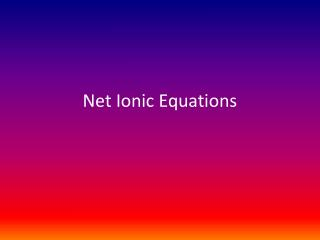DownloadDownload PresentationNet Ionic Equations

# Net Ionic Equations

Télécharger la présentation## Net Ionic Equations

- - - - - - - - - - - - - - - - - - - - - - - - - - - E N D - - - - - - - - - - - - - - - - - - - - - - - - - - -
##### Presentation Transcript

1. Net Ionic Equations

2. Net Ionic Equations: Used for Double Displacement Reactions Show Only the Ions that are involved in the reaction! - Ionic substances that are (aq) - show ions separated - Ionic substances that are (s )- show together Complete Ionic Equation - Shows all ions. Net Ionic Equation - Cancel out ions present on both sides of arrow. - These ions are called spectator ions.

3. NaBr(s) + RbCl(s) --> NaCl(s) + RbBr(s) • NO REACTION

4. Combine solutions of sodium carbonate and calcium nitrate

5. Write the ionic and net ionic equation:KCl(aq) + AgNO3 (aq) --> KNO3 (aq) + AgCl (s)

6. Write the ionic and net ionic equationLi2S(aq) + Ni(NO3)2(aq) --> LiNO3 (aq) + NiS (s)

7. Write the ionic and net ionic equation Pb(NO3)2(aq) + KCl(aq) --> Al(NO3)3(aq) + Ba(OH)2(aq) -->